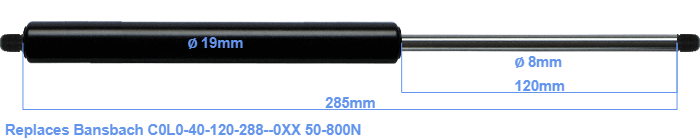# Replacement for Bansbach C0L0-40-120-288–0XX 50-800N

\$29.29

Replacement gas spring for the Bansbach C0L0-40-120-288--0XX 50-800 Newton. Thread: M8. Brand: Stabilus Industry Line.
 Force Choose an option50 Newton60 Newton80 Newton100 Newton120 Newton140 Newton150 Newton160 Newton180 Newton200 Newton220 Newton240 Newton250 Newton260 Newton280 Newton300 Newton320 Newton340 Newton350 Newton360 Newton380 Newton400 Newton420 Newton440 Newton450 Newton460 Newton480 Newton500 Newton520 Newton540 Newton550 Newton560 Newton580 Newton600 Newton620 Newton640 Newton650 Newton660 Newton680 Newton700 Newton720 Newton740 Newton750 Newton760 Newton780 Newton800 NewtonClearThe diameter of the cylinder of this gas spring is 19 millimeters. The diameter of the rod is 8 millimeter. The stroke is 120 millimeter long. The total length of this replacement gas spring is 285 mm. This is the total distance between the centers of the two mounting parts. The length from thread to thread (wihout mounting parts) is 285 mm. This replacement gas spring has a force of 50-800 Newton. This is not an official Bansbach gas spring, but a so called replacement. HAHN Gasfedern, a Stabilus subsidiary, is responsible for the production of this replacement gas spring. The gas spring has the brand Stabilus Industry Line. The gas spring can be used without problems as a replacement, because the dimensions and force equal.